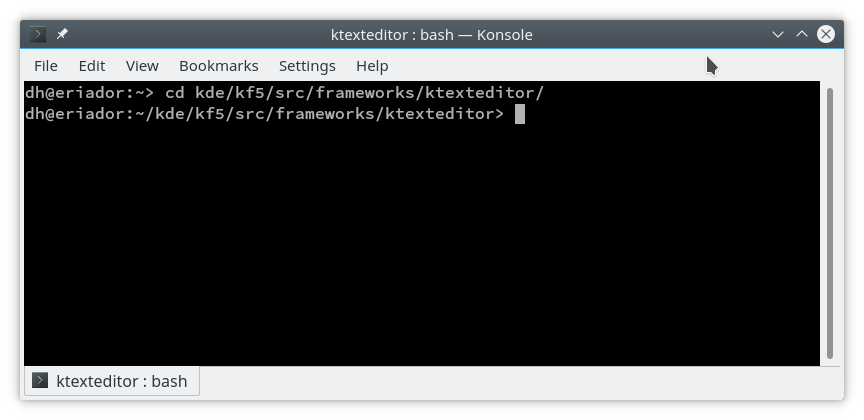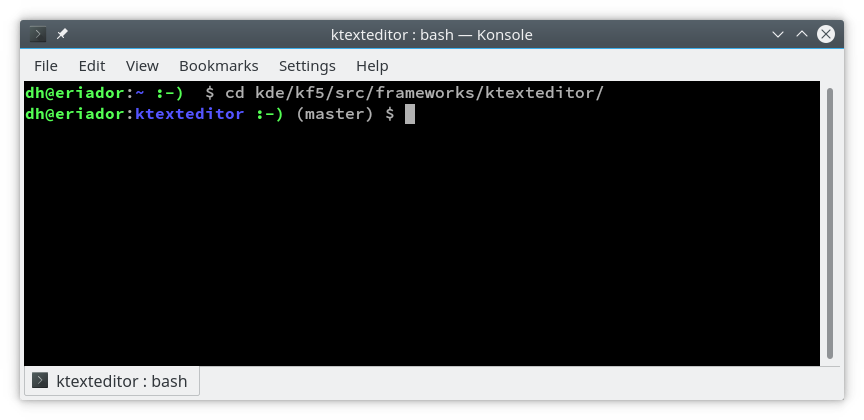# Fancy Terminal Prompt

By default, the terminal looks as follows on my Linux distribution:However, if you are working a lot on the terminal, there are a lot of scripts and tricks available in the net that improve the information displayed in the terminal in many ways. For instance, since many many years, I have the following at the end of my ~/.bashrc:

# use a fancy prompt
PS1="$\033[01;32m$\u@\h$\033[00m$:$\033[01;34m$\W$\033[00m$"
PS1="$PS1 \if [ \$? = 0 ]; then echo -e '$\033[01;32m$:-)';"
PS1="$PS1 else echo -e '$\033[01;31m$:-(' \$?; fi\$\033[00m$"
PS1="$PS1 \$(__git_ps1 \"(%s)\") \\$ "

Once you open a new terminal, then the appearance is as follows:As you can see, now I have nice colors: The hostname is green, the folder is blue, the return value of the last executed command is a green :-) in case of success (exit code = 0), and a red :-( in case of errors (exit code != 0). In addition, the last part shows the current git branch (master). Showing the git branch is very useful especially if you are using arc a lot and work with many branches.

I am sure there are many more cool additions to the terminal. If you have some nice additions, please share – maybe also as a new blog?